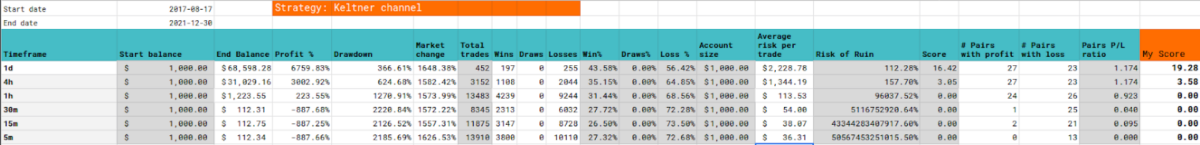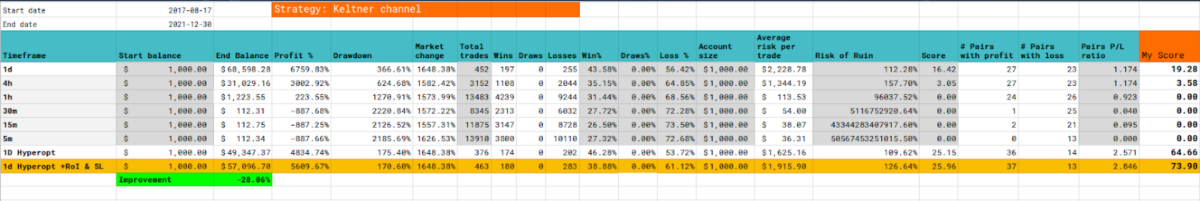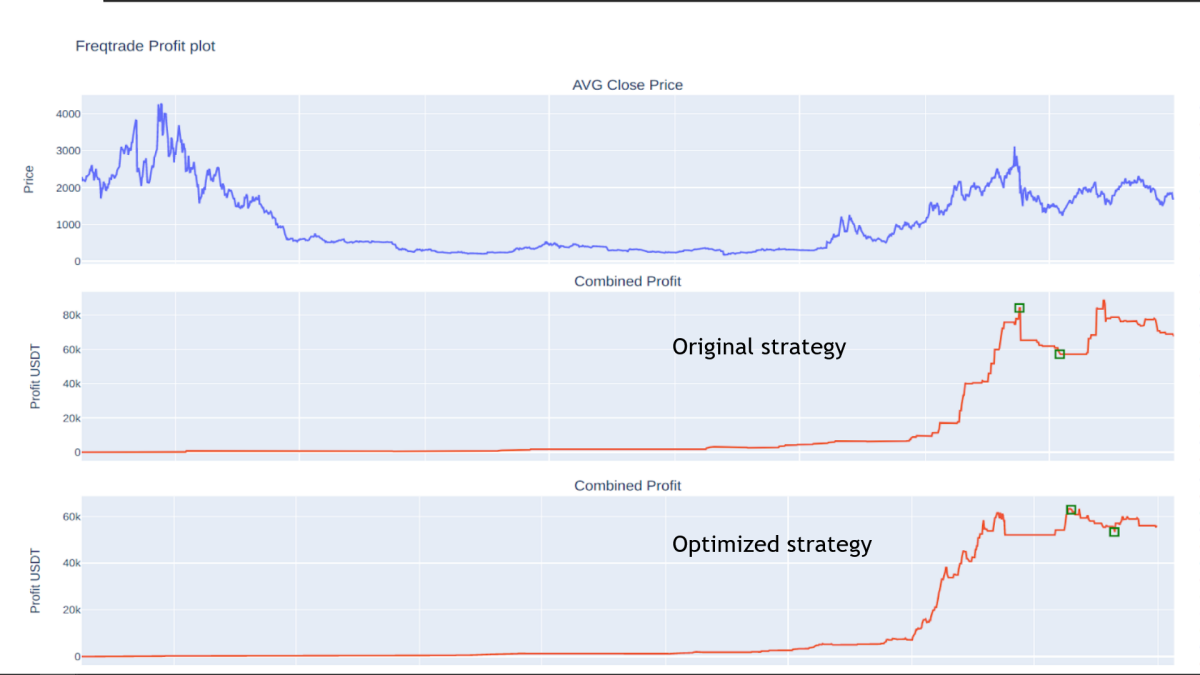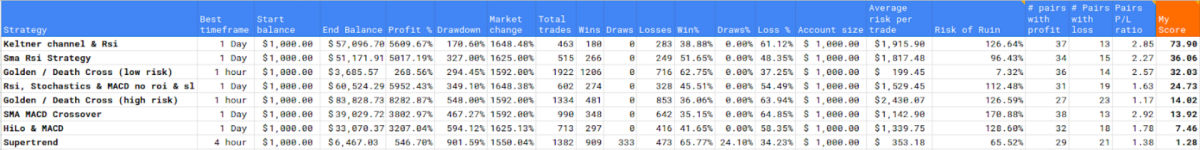# Keltner channel & Rsi strategy - Is this strategy profitable or not?

### Intro

In this blog series I will be searching for the best trading strategy for cryptocurrency trading with a trading bot. These posts are complementary to the video's I make on Youtube, which you can watch below.

The trading bot I use is the Freqtrade (https://www.freqtrade.io/en/latest/) trading bot which not only has good options for bot trading, but also is excellent in backtesting and optimization of trading parameters. Therefore I will use this program to not only trade automatically, but also look for the best strategies and setups.

All code that is specifically written for this strategy or for hyperparameter optimisation is available at the bottom of this post.

If you appreciate the effort I take to create this strategy or optimisation code, then please consider a tip for this post!

### The backtesting setup

If you want to know more on how I do these backtests like: which pairs I use, which timeframes and which periods I test, then see this blog post to know more about the approach I use: https://www.publish0x.com/dutchcryptodad/is-it-profitable-or-not-the-setup-and-approach-xppgjoj

### Strategy

In this post I will show you the results of the backtests I did on the "Keltner Channel Strategy".

This strategy makes use of the Keltner channel, as my primary indicator and the RSI as my confirmation indicator. The Keltner channel is a volatility based band around a simple moving average. It uses the average true range to determine the upper and lower band. These bands will act like some form of support and resistance.

I will not discuss the Keltner channel in much detail because there are other excellent internet resources that can provide you with a great deal of information (see Investopedia). This strategy is quite simple but effective. It is a trend following strategy which uses only two signals to trigger a buy and one signal to trigger a sell.

When the price of an asset closes above the upper band of the Keltner channel and the RSI is at least at a level of 55, then a buy signal occurs. This indicates that there is enough momentum on the asset to attain higher prices. If you know Bollinger Bands, then you will recognise this immediately and you can compare this as the riding the bands strategy.

The sell signal occurs when the price closes below the moving average line and this indicates that the trend is ending.

### Stoploss and takeprofit

This strategy does not use the default Freqtrade ROI and Stoploss but solely depends on the entry and exit signals from the indicators described above.

### Initial backtest results

After backtesting, the initial backtest results are as follows:

• Best timeframe: 1 day
• Total profit of strategy: 6760 %
• Drawdown of strategy: 366 %
• Winrate 43 %
• Risk of ruin of strategy: 112### Hyperparameter optimisation

In the Hyperopt session I wil ltry to find the optimal settings for the following parameters:

• The Simple moving average range
• The ATR range for the Keltner band
• The RSI Buy horizontal line treshold

After this session, there will be another backtest with the optimised parameters on the given timeframe and range to see if this strategy has improved or not.

### Conclusions

Interestingly enough, this time parameter optimisation did not seem to find a better setup than the original strategy has. On almost all aspects the performance decreased. Except the drawdown of the strategy seemed to have improved a lot.

Apparently we already use the most optimal settings for this strategy at this moment.What I can see in the original strategy is that is has some very steep runs and then form a plateau where there is hardly any profit. The biggest drawdown happens at the beginning of may 2021 and that’s where the initial bull wave ended that started after the covid crash in march 2020.

The optimized strategy has a much smoother curve with some small hooks. And what strikes me is that this strategy did not put on trades after the initial bull wave. And that’s exactly where the original strategy got its biggest drawdown from.
Also this strategies drawdown started in oktober 2021 and ended in november 2021.

Finally this whole section of the optimized strategy looks more of a plateau and looks good to me to form a new level for upwards movement.

### Strategy League

Nonetheless, even though the strategies performance did not improve after optimisation, it still looks to be a very profitable one in comparison to the earlier tested strategies. As you can see on the image below, it actually is the new number one strategy at this time.### The strategy code

``````# --- Do not remove these libs ---
from pandas import DataFrame

import talib.abstract as ta
import pandas_ta as pta
import numpy as np  # noqa
import pandas as pd  # noqa

# These libs are for hyperopt
from functools import reduce
from freqtrade.strategy import (BooleanParameter, CategoricalParameter, DecimalParameter,IStrategy, IntParameter)

class keltnerchannel(IStrategy):
timeframe = "1d"
# Both stoploss and roi are set to 100 to prevent them to give a sell signal.
stoploss = -100
minimal_roi = {"0": 100}

plot_config = {
"main_plot": {
"kc_upperband" : {"color": "purple",'plotly': {'opacity': 0.4}},
"kc_middleband" : {"color": "blue"},
"kc_lowerband" : {"color": "purple",'plotly': {'opacity': 0.4}}
},
"subplots": {
"RSI": {
"rsi": {"color": "orange"},
"hline": {"color": "grey","plotly": {"opacity": 0.4}}
},
},
}

def populate_indicators(self, dataframe: DataFrame, metadata: dict) -> DataFrame:
# Keltner Channel
keltner = qtpylib.keltner_channel(dataframe, window=20, atrs=1)
dataframe["kc_upperband"] = keltner["upper"]
dataframe["kc_lowerband"] = keltner["lower"]
dataframe["kc_middleband"] = keltner["mid"]

# RSI
dataframe["rsi"] = ta.RSI(dataframe, timeperiod=14)

# Horizontal RSI line
hline = 55
dataframe['hline'] = hline

# Print stuff for debugging dataframe
# print(dataframe.tail(20))
return dataframe

dataframe.loc[
(qtpylib.crossed_above(dataframe['close'], dataframe['kc_upperband'])
& (dataframe["rsi"] > dataframe['hline'])
),

] = 1

return dataframe

def populate_sell_trend(self, dataframe: DataFrame, metadata: dict) -> DataFrame:
dataframe.loc[
(qtpylib.crossed_below(dataframe['close'], dataframe['kc_middleband'])),

"sell",
] = 1
return dataframe``````

### The hyperopt code

``````# --- Do not remove these libs ---
from pandas import DataFrame

import talib.abstract as ta
import pandas_ta as pta
import numpy as np  # noqa
import pandas as pd  # noqa

# These libs are for hyperopt
from functools import reduce
from freqtrade.strategy import (BooleanParameter, CategoricalParameter, DecimalParameter,IStrategy, IntParameter)

class keltnerhopt(IStrategy):
timeframe = "1d"
# Both stoploss and roi are set to 100 to prevent them to give a sell signal.
stoploss = -100
minimal_roi = {"0": 100}

# Hyperopt spaces
window_range = IntParameter(13, 56, default=16, space="buy")
atrs_range = IntParameter(1, 8, default=1, space="buy")

def populate_indicators(self, dataframe: DataFrame, metadata: dict) -> DataFrame:
# Keltner Channel
for windows in self.window_range.range:

# Rsi
dataframe["rsi"] = ta.RSI(dataframe, timeperiod=14)

# Print stuff for debugging dataframe
# print(dataframe.tail(20)
return dataframe

conditions = []
conditions.append(
(qtpylib.crossed_above(dataframe['close'], dataframe[f"kc_upperband_{self.window_range.value}_{self.atrs_range.value}"]))
)

if conditions:
dataframe.loc[
reduce(lambda x, y: x & y, conditions),

return dataframe

def populate_sell_trend(self, dataframe: DataFrame, metadata: dict) -> DataFrame:
conditions = []
conditions.append(
(qtpylib.crossed_below(dataframe['close'], dataframe[f"kc_middleband_{self.window_range.value}_{self.atrs_range.value}"]))
)

if conditions:
dataframe.loc[
reduce(lambda x, y: x & y, conditions),
'sell'] = 1

return dataframe``````

### The hyperopt json results

``````{
"strategy_name": "keltnerhopt",
"params": {
"trailing": {
"trailing_stop": false,
"trailing_stop_positive": null,
"trailing_stop_positive_offset": 0.0,
"trailing_only_offset_is_reached": false
},
"atrs_range": 1,
"window_range": 16
},
"sell": {},
"protection": {},
"roi": {
"0": 0.696,
"10216": 0.40800000000000003,
"26070": 0.143,
"41881": 0
},
"stoploss": {
"stoploss": -0.254
}
},
"ft_stratparam_v": 1,
"export_time": "2022-02-02 17:39:05.540085+00:00"
}``````I'm just a regular Dutch dad with a passion for crypto, trading, technology and learning. This channel is my personal journey into the world of Crypto, blockchain, programming, trading bots, trading strategies, NFT's, Defi and many things more.